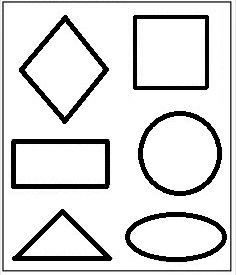Home > Kindergarten > Geometry > Analyzing Shapes

# Analyzing Shapes

Directions: Using the diagram, fill in the blanks with the names of the shapes to make each statement true.__________ has more sides than __________

__________ has the same number of sides as __________

__________ has more vertices than __________

### Hint

What do you know about each shape?

How many sides to they have?

How many vertices? (vertices are the points at corners (where two sides meet))

There are various possible answers, one is:
The triangle has more sides than the oval (ellipse).
The rhombus (or kite or diamond) has the same number of sides as the square.
The rectangle has more vertices than the circle.

Source: Bryan Anderson

## Adding and Subtracting Within 10

Directions: Using the digits 1 to 9 at most one time each, place a digit …

1.Why do kindergartners do GEOMETRY?!?!?!?!?

2.Calvin Petruccelli

1. Diamond has more sides than circle

2. Diamond has the same amount of sides as a rectangle.

3. A rectangle has more vertices than a triangle.

3.square,triangle
diamond,rectangle
triangle,circle

4.Rhombus has more sides than oval
Rectangle has the same number of sides as square

Triangle has more vertices than Circle

•A square has more sides then triangle.
A square and rectangle have the same number of sides.
A triangle has more vertices then circle.

5.______rectangle____ has more sides than ____circle______

___square_______ has the same number of sides as ____rectangle______

_____square_____ has more vertices than _____triangle_____

6.a square has more sides than a triangle
a rectangle has the same number of sides as a square

7.rectangle, triangle
rectangle, square
rhombus, triangle

8.rectangle has more sides than a circle
diamond has the same amount of sides as square
triangle has more vertices than oval

9.Avery Cweklinski

Diamond has more sides than a triangle
Rectangle has the same as a square
Triangle has more vertices than a circle

10.a rectangle has more sides than a triangle.
a square and a rectangle have the same number of sides.
triangle has more vertices than a circle

11.rectangle, triangle
square,rectangle
diamond, triangle

12.1) Diamond has more sides than Triangle
2)Square , Diamond, has the same no.of sides as Rectangle.
3)Diamond has more vertics than Circle and Oval.

13.diamond, triangle
square, rectangle
triangle, circle

14.The diamond has more sides than the circle.

The rectangle has the same number of sides as the square.

The square has more vertices than the triangle.

🙂 🙂 🙂

15.16.17.I finaly learned to do emojies! 🙂 🙁 ;);(